In Online Education NCERT Solutions for Class 7 Maths Chapter 9 Rational Numbers Ex 9.1 are part of NCERT Solutions for Class 7 Maths. Here we have given NCERT Solutions for Class 7 Maths Chapter 9 Rational Numbers Ex 9.1.

 Board CBSE Textbook NCERT Class Class 7 Subject Maths Chapter Chapter 9 Chapter Name Rational Numbers Exercise Ex 9.1 Number of Questions Solved 10 Category NCERT Solutions

## Online Education NCERT Solutions for Class 7 Maths Chapter 9 Rational Numbers Ex 9.1

Question 1.
List five rational numbers between

1. -1 and 0
2. -2 and -1
3. –$$\frac { 4 }{ 5 }$$ and – $$\frac { 2 }{ 3 }$$
4. –$$\frac { 1 }{ 2 }$$ and $$\frac { 2 }{ 3 }$$

Solution: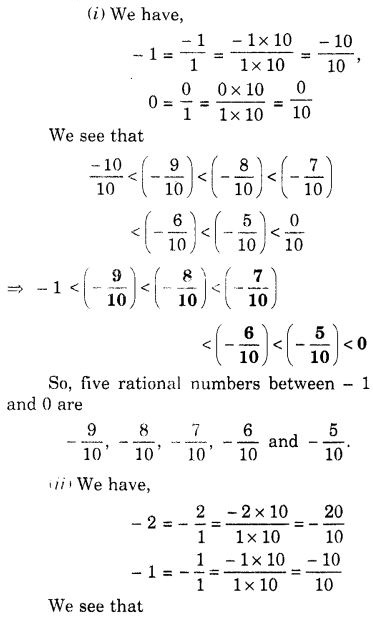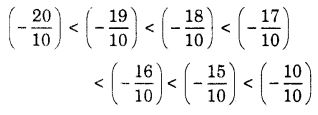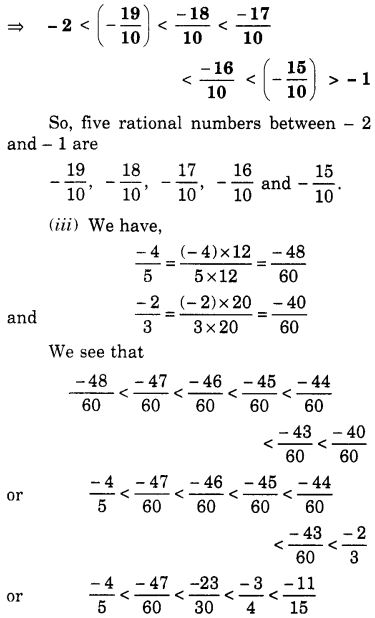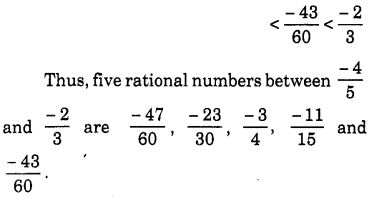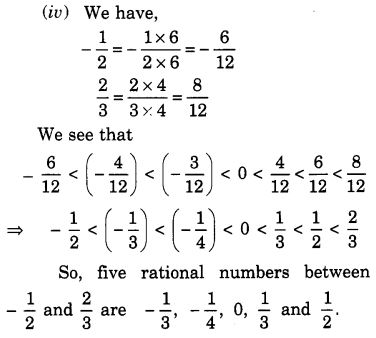Question 2.
Write four more rational numbers in each of the following patterns :Solution: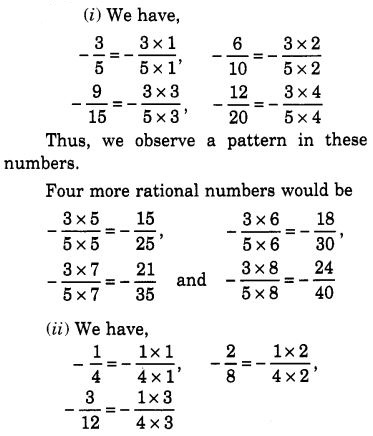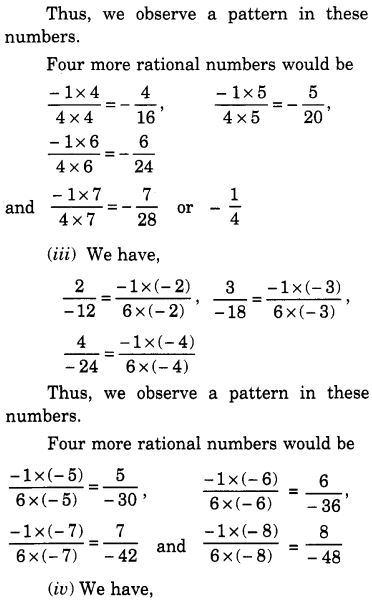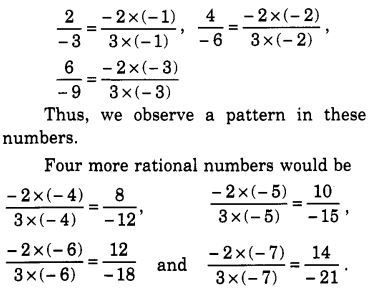Question 3.
Give four rational numbers equivalent to:
(i) $$\frac { -2 }{ 7 }$$
(ii) $$\frac { 5 }{ -3 }$$
(iii) $$\frac { 4 }{ 9 }$$

Solution:
(i) Four rational numbers equivalent to $$\frac { -2 }{ 7 }$$ are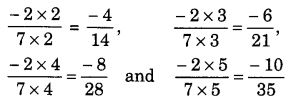(ii) Four rational numbers equivalent to $$\frac { 5 }{ -3 }$$ are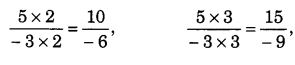(iii) Four rational numbers equivalent to $$\frac { 4 }{ 9 }$$ areQuestion 4.
Draw the number line and represent the following rational numbers on it:

1. $$\frac { 3 }{ 4 }$$
2. $$\frac { -5 }{ 8 }$$
3. $$\frac { -7 }{ 4 }$$
4. $$\frac { 7 }{ 8 }$$

Solution:Question 5.
The points P, Q, R, S, T, U, A, and B on this number line are such that, TR = RS = SU and AP = PQ = QB. Name the rational numbers represented by P, Q, R, and S.Solution:
The rational number represented by P is 2 $$\frac { 1 }{ 3 }$$ = $$\frac { 7 }{ 3 }$$
The rational number represented by Q is 2 $$\frac { 2 }{ 3 }$$ = $$\frac { 8 }{ 3 }$$
The rational number represented by R is -1 $$\frac { 1 }{ 3 }$$ = $$\frac { -4 }{ 3 }$$
The rational number represented by S is -1 $$\frac { 2 }{ 3 }$$ = $$\frac { -5 }{ 3 }$$
The rational numbers represented by P, Q, R and S are 2 $$\frac { 1 }{ 3 }$$, 2 $$\frac { 2 }{ 3 }$$, -1 $$\frac { 1 }{ 3 }$$ and – 1 $$\frac { 2 }{ 3 }$$ respectively

Question 6.
Which of the following pairs represent the same rational number?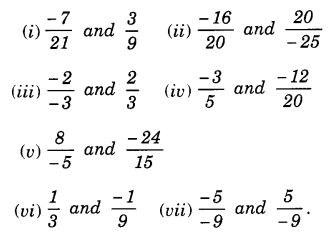Solution:
(i) $$\frac { -7 }{ 21 }$$ is a negative rational number and $$\frac { 3 }{ 9 }$$ is a positive rational number. So, the given pair does not represent the same rational number.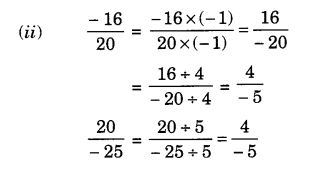So, the given pair represents the same rational number.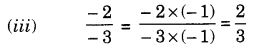So, the given pair represents the same rational number.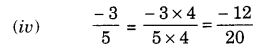So, the given pair represents the same rational number.So, the given pair represents the same rational number.
(vi) $$\frac { 1 }{ 3 }$$ is a positive rational number and $$\frac { -1 }{ 9 }$$ is a negative rational number. So, the given pair does not present the same rational number.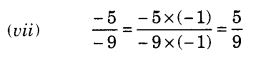Thus, $$\frac { -5 }{ -9 }$$ is a positive rational number and $$\frac { 5 }{ -9 }$$ is a negative rational number. So, the given pair does not represent the same rational number.

Question 7.
Rewrite the following rational numbers in the simplest form :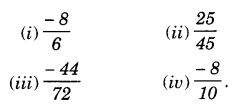Solution: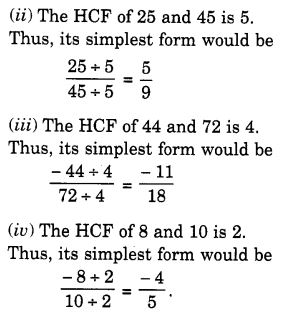Question 8.
Fill in the boxes with the correct symbol out of >, < and =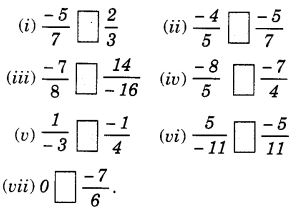Solution: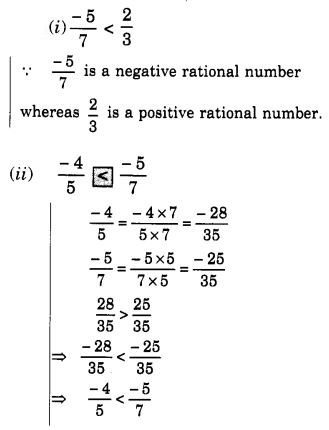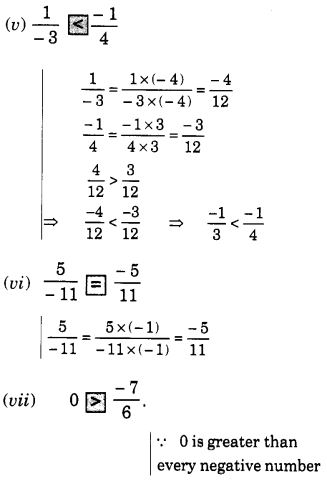Question 9.
Which is greater in each of the following?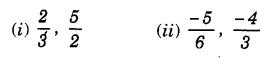Solution:Question 10.
Write the following rational numbers in ascending order :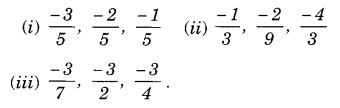Solution: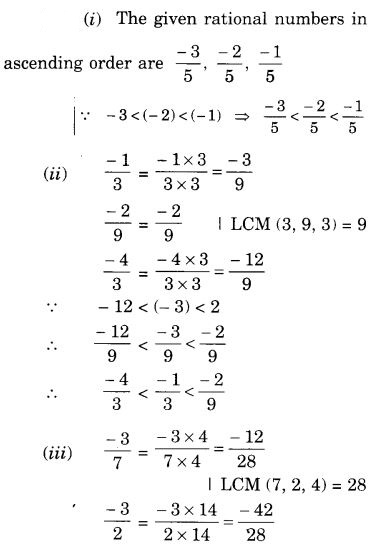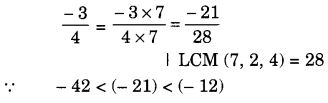We hope the NCERT Solutions for Class 7 Maths Chapter 9 Rational Numbers Ex 9.1 help you. If you have any query regarding NCERT Solutions for Class 7 Maths Chapter 9 Rational Numbers Ex 9.1, drop a comment below and we will get back to you at the earliest.